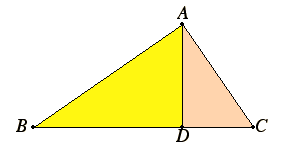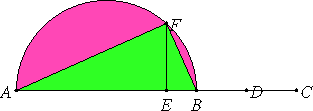# Lemma

Let ABC be a right-angled triangle having the angle A right, and let the perpendicular AD be drawn.

I say that the rectangle CB by BD equals the square on BA, the rectangle BC by CD equals the square on CA, the rectangle BD by DC equals the square on AD, and the rectangle BC by AD equals the rectangle BA by AC, and first that the rectangle CB by BD equals the square on BA.VI.8

Since in a right-angled triangle AD has been drawn from the right angle perpendicular to the base, therefore the triangles ABD and ADC are similar both to the whole ABC and to one another.

Since the triangle ABC is similar to the triangle ABD, therefore CB is to BA as BA is to BD. Therefore the rectangle CB by BD equals the square on AB. For the same reason the rectangle BC by CD also equals the square on AC.

Since, if in a right-angled triangle a perpendicular is drawn from the right angle to the base, then the perpendicular so drawn is a mean proportional between the segments of the base, therefore BD is to DA as AD is to DC. Therefore the rectangle BD by DC equals the square on AD.

I say that the rectangle BC by AD also equals the rectangle BA by AC.

VI.4

Since we said, ABC is similar to ABD, therefore BC is to CA as BA is to AD.

Therefore the rectangle BC by AD equals the rectangle BA by AC.

Q.E.D.

# Proposition 33

To find two straight lines incommensurable in square which make the sum of the squares on them rational but the rectangle contained by them medial.
X.30

Set out two rational straight lines AB and BC commensurable in square only such that the square on the greater AB is greater than the square on the less BC by the square on a straight line incommensurable with AB.VI.28

Bisect BC at D. Apply to AB a parallelogram equal to the square on either of the straight lines BD or DC and deficient by a square figure, and let it be the rectangle AE by EB.

Describe the semicircle AFB on AB, draw EF at right angles to AB, and join AF and FB.

X.18

Since AB and BC are unequal straight lines, and the square on AB is greater than the square on BC by the square on a straight line incommensurable with AB, while there was applied to AB a parallelogram equal to the fourth part of the square on BC, that is, to the square on half of it, and deficient by a square figure, making the rectangle AE by EB, therefore AE is incommensurable with EB.

And AE is to EB as the rectangle BA by AE is to the rectangle AB by BE, while the rectangle BA by AE equals the square on AF, and the rectangle AB by BE is to the square on BF, therefore the square on AF is incommensurable with the square on FB. Therefore AF and FB are incommensurable in square.

I.47

Since AB is rational, therefore the square on AB is also rational, so that the sum of the squares on AF and FB is also rational.

Since, again, the rectangle AE by EB equals the square on EF, and, by hypothesis, the rectangle AE by EB also equals the square on BD, therefore FE equals BD. Therefore BC is double FE, so that the rectangle AB by BC is also commensurable with the rectangle ABEF.

But the rectangle AB by BC is medial,
therefore the rectangle AB by EF is also medial.

Lemma

But the rectangle AB by EF equals the rectangle AF by FB, therefore the rectangle AF by FB is also medial.

But it was also proved that the sum of the squares on these straight lines is rational.

Therefore two straight lines AF and FB incommensurable in square have been found which make the sum of the squares on them rational, but the rectangle contained by them medial.

Q.E.D.

## Guide

The first part of the lemma encompasses proposition I.47, but the proof of it depends on the theory of similar triangles developed in Book VI, unlike Euclid’s proof of I.47.

This proposition is used in propositions X.39 and X.76.